# Smart Health Care Monitoring System Based on IoT

This project includes hardware which will measure blood pressure, heart rate, ECG & temperature, and send to Android application & Firebase.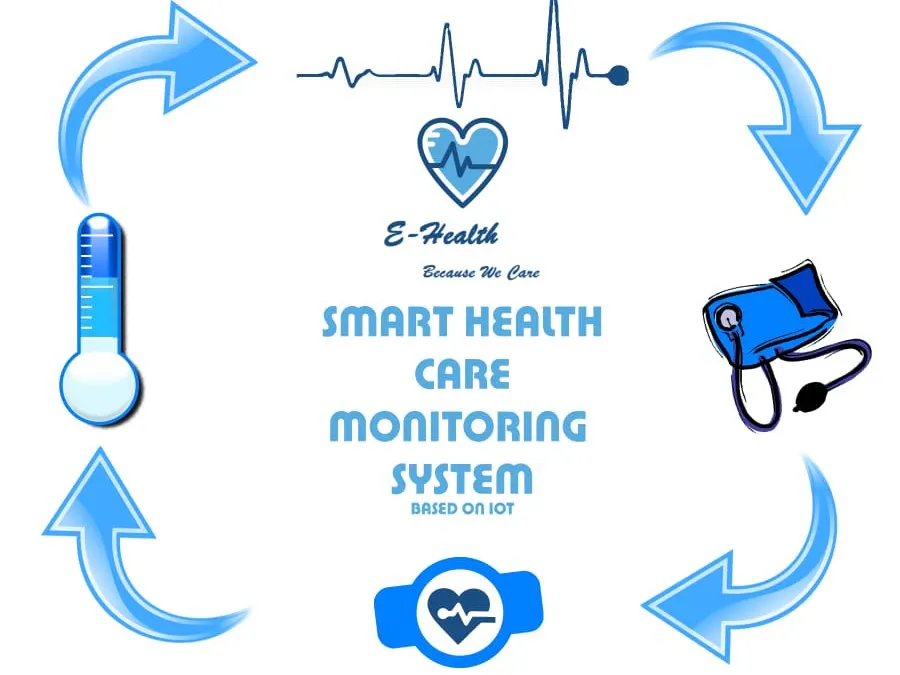## Things used in this project

### Hardware componentsArduino UNO & Genuino UNO
×1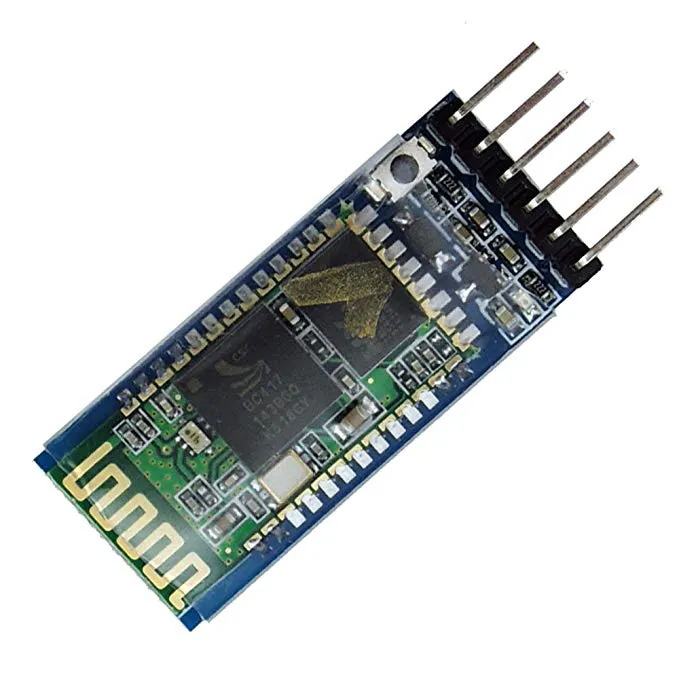HC-05 Bluetooth Module
×1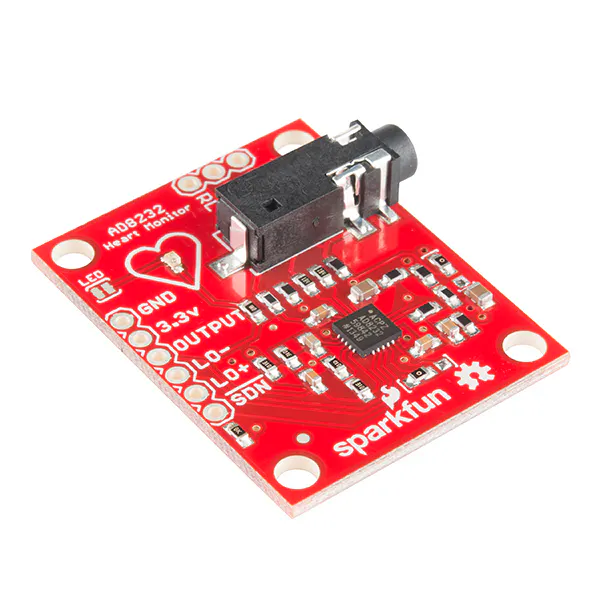×1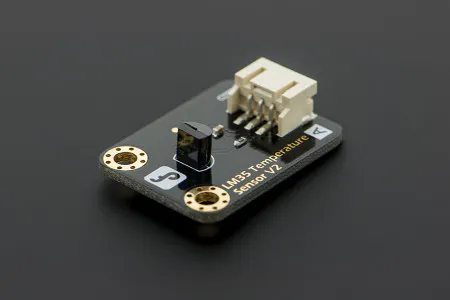DFRobot Gravity: Analog LM35 Temperature Sensor For Arduino
×1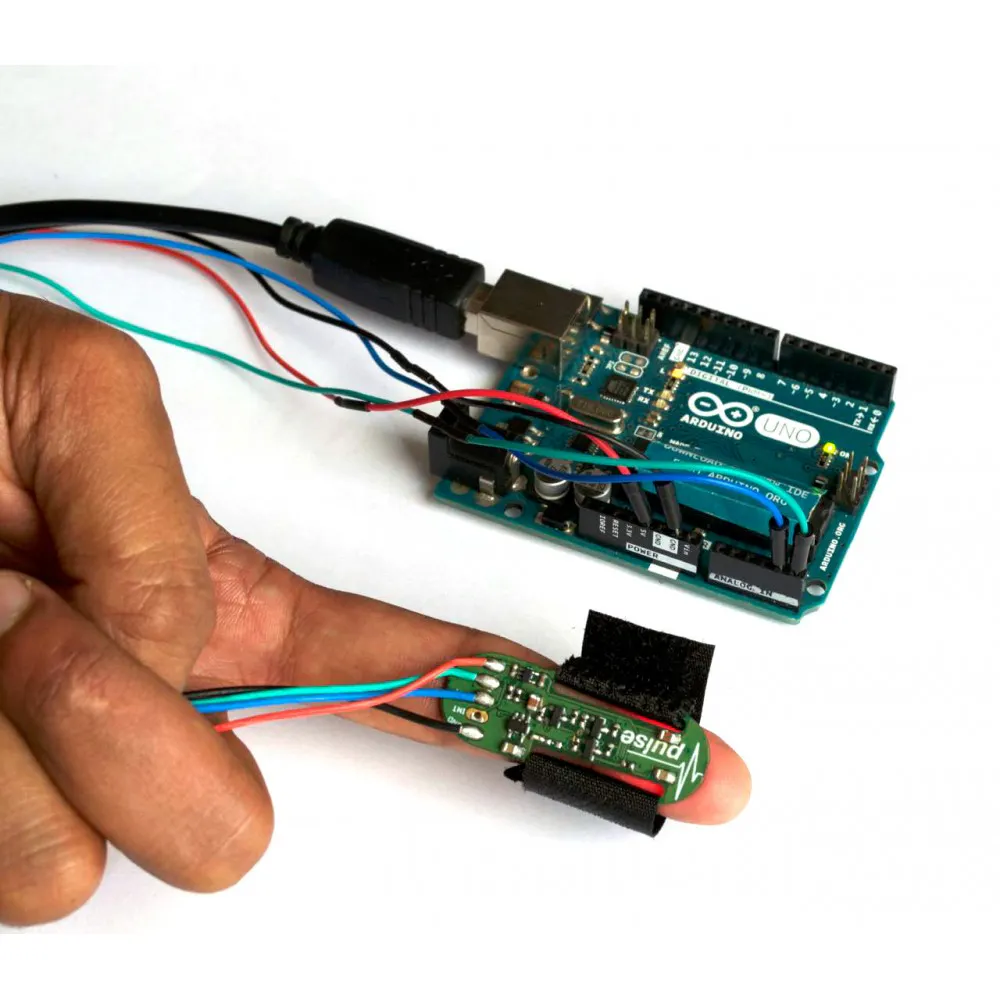ProtoCentral Pulse Oximeter & Heart Rate Sensor based on MAX30100
×1×1
 Sunrom Blood Preasure Module
×1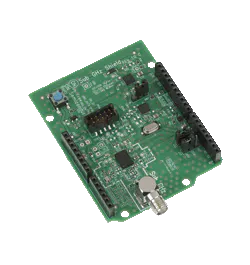ON Semiconductor EU-SIGFOX-GEVB
×1

### Software apps and online servicesAndroid StudioArduino IDE### Hand tools and fabrication machines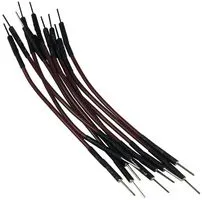10 Pc. Jumper Wire Kit, 5 cm Long

## Schematics

### Block Diagram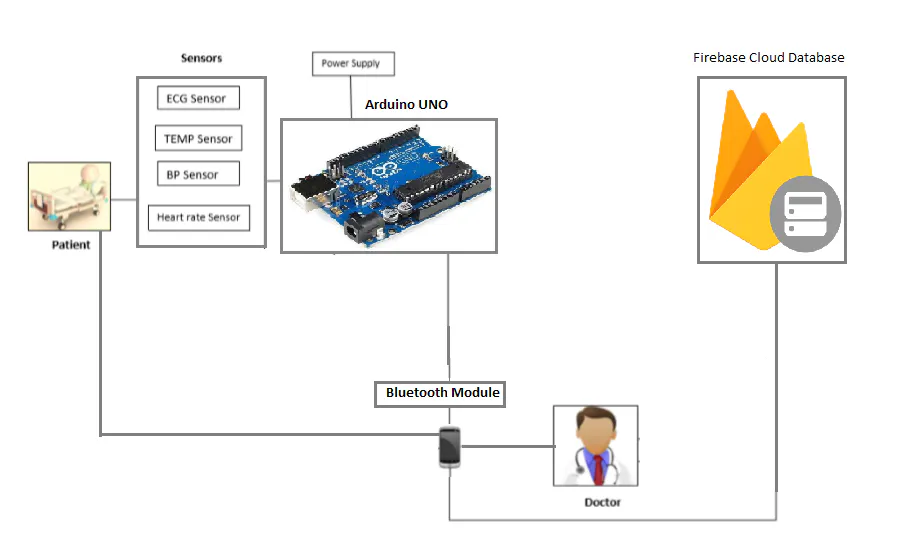## Code

### Arduino Code

Arduino
```#define USE_ARDUINO_INTERRUPTS true
#include <PulseSensorPlayground.h>
#include <SoftwareSerial.h>

SoftwareSerial mySerial(10, 11); // RX, TX
const int PulseWire = 0;
int tempPin = 1;
int ECGPin = 2;
String incomingByte;
int value;
int myBPM =0;
int finalBPM ;
float volts=0.0;
float cel =0;
String stringTemp;
String stringBPM;
bool is_data_send=false;
bool ISBP=false;
bool ISECG=false;
bool ISHR=false;
bool IStemp=false;
const int LED13 = 13;
int Threshold = 550;
String data="";

PulseSensorPlayground pulseSensor;

void setup() {

Serial.begin(38400);
Serial.println("Enter AT commands:");
mySerial.begin(38400);

pulseSensor.setThreshold(Threshold);

if (pulseSensor.begin()) {
Serial.println("We created a pulseSensor Object !");
}
}

void loop() {

if (mySerial.available()) {

Serial.println(incomingByte);

if(incomingByte=="49")
{ISECG=false;ISBP=false;ISHR=false;IStemp=true;is_data_send=true;}
else if(incomingByte=="50")
{ISECG=false;ISBP=false;ISHR=true;IStemp=false;is_data_send=true;}
else if(incomingByte=="51")
{ISECG=false;ISBP=false;ISHR=true;IStemp=true;is_data_send=true;}
else if(incomingByte=="52")
{ISECG=false;ISBP=true;ISHR=false;IStemp=false;is_data_send=true;}
else if(incomingByte=="53")
{ISECG=false;ISBP=true;ISHR=false;IStemp=true;is_data_send=true;}
else if(incomingByte=="54")
{ISECG=false;ISBP=true;ISHR=true;IStemp=false;is_data_send=true;}
else if(incomingByte=="55")
{ISECG=false;ISBP=true;ISHR=true;IStemp=true;is_data_send=true;}
else if(incomingByte=="56")
{ISECG=true;ISBP=false;ISHR=false;IStemp=false;is_data_send=true;}
else if(incomingByte=="57")
{ISECG=true;ISBP=false;ISHR=false;IStemp=true;is_data_send=true;}
else if(incomingByte=="65")
{ISECG=true;ISBP=false;ISHR=true;IStemp=false;is_data_send=true;}
else if(incomingByte=="66")
{ISECG=true;ISBP=false;ISHR=true;IStemp=true;is_data_send=true;}
else if(incomingByte=="67")
{ISECG=true;ISBP=true;ISHR=false;IStemp=false;is_data_send=true;}
else if(incomingByte=="68")
{ISECG=true;ISBP=true;ISHR=false;IStemp=true;is_data_send=true;}
else if(incomingByte=="69")
{ISECG=true;ISBP=true;ISHR=true;IStemp=false;is_data_send=true;}
else if(incomingByte=="70")
{ISECG=true;ISBP=true;ISHR=true;IStemp=true;is_data_send=true;}

}
//Getting Values from Sensors
if(is_data_send)
{
int counter=0;
if(IStemp||ISHR){
while(counter<10){
if(IStemp){
volts=(value/1024.0)*5.0;      //conversion to volts
cel = cel+(volts*100.0);
}
if(ISHR){
if (pulseSensor.sawStartOfBeat()) {
myBPM = myBPM+pulseSensor.getBeatsPerMinute();
}
}

counter=counter+1;
}}
if(ISBP){
}
int counter2=0;
if(ISECG){
while(counter2<500){
else{
Serial.println(abc);
data+=abc+"|";
}
counter2=counter2+1;
}
}
}
//Sending Data
if(is_data_send){
if(ISBP){
}
if(ISHR){
float final_bpm=myBPM/10;
stringBPM = String(final_bpm);
data+=stringBPM+"|";
}
if(IStemp){
float final_temp=cel/10;
stringTemp = String(final_temp);
data+=stringTemp+"|";
}

Serial.print("Data sending");
Serial.print(data);
//Serial.print(stringTemp+"|"+stringBPM);
mySerial.print(data);
mySerial.println();
is_data_send=false;
}
delay(20);                    // considered best practice in a simple sketch.

}

```

## Credits

### Muneeb Abdul Rauf

0 projects • 4 followers
Thanks to Anatoliy.